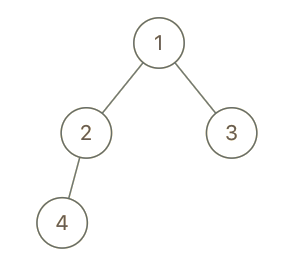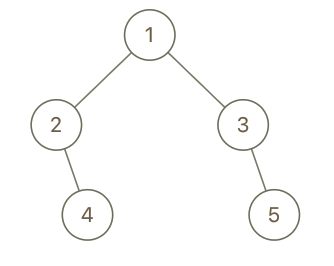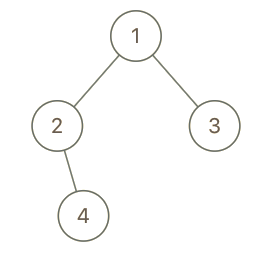Tags: "leetcode", "binary-tree", "dfs", access_time 2-min read

# Cousins in Binary Tree

#### Created: May 7, 2020 by [lek-tin]

##### Last updated: May 7, 2020

In a binary tree, the root node is at depth `0`, and children of each depth k node are at depth `k+1`.

Two nodes of a binary tree are cousins if they have the same depth, but have different parents.

We are given the `root` of a binary tree with unique values, and the values `x` and `y` of two different nodes in the tree.

Return `true` if and only if the nodes corresponding to the values `x` and `y` are cousins.

### Example 1:``````Input: root = [1,2,3,4], x = 4, y = 3
Output: false
``````

### Example 2:``````Input: root = [1,2,3,null,4,null,5], x = 5, y = 4
Output: true
``````

### Example 3:``````Input: root = [1,2,3,null,4], x = 2, y = 3
Output: false
``````

#### Note:

1. The number of nodes in the tree will be between `2` and `100`.
2. Each node has a unique integer value from `1` to `100`.

### Solution

Java

``````/**
* Definition for a binary tree node.
* public class TreeNode {
*     int val;
*     TreeNode left;
*     TreeNode right;
*     TreeNode() {}
*     TreeNode(int val) { this.val = val; }
*     TreeNode(int val, TreeNode left, TreeNode right) {
*         this.val = val;
*         this.left = left;
*         this.right = right;
*     }
* }
*/
class Solution {
boolean shared_parent = false;
int x_level = -1;
int y_level = -1;

public boolean isCousins(TreeNode root, int x, int y) {
dfs(root, x, y, 0);
System.out.println(shared_parent);
if (x_level > 0 && y_level > 0 && x_level == y_level && !shared_parent) {
return true;
}

return false;
}

private void dfs(TreeNode root, int x, int y, int level) {
if (root == null) return;

// early stop
if (shared_parent) return;
if (x_level > 0 && y_level > 0 && x_level != y_level) return

if (root.val == x) {
x_level = level;
return;
}
if (root.val == y) {
y_level = level;
return;
}

if  (root.left != null && root.right != null) {
System.out.println(root.left.val + ", " + root.right.val);
if ( (root.left.val == x && root.right.val == y) ||
(root.left.val == y && root.right.val == x)
) {
shared_parent = true;
return;
}
}

dfs(root.left, x, y, level+1);
dfs(root.right, x, y, level+1);
}
}
``````